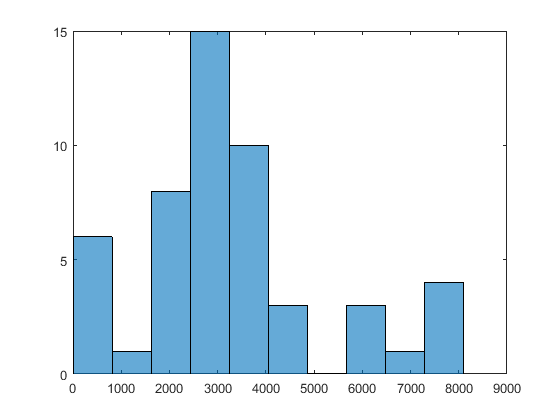## Multiple Solutions

You obtain multiple solutions in an object by calling `run` with the syntax

`[x,fval,exitflag,output,manymins] = run(...);`

`manymins` is a vector of solution objects; see `GlobalOptimSolution`. The `manymins` vector is in order of objective function value, from lowest (best) to highest (worst). Each solution object contains the following properties (fields):

There are several ways to examine the vector of solution objects:

• In the MATLAB® Workspace Browser. Double-click the solution object, and then double-click the resulting display in the Variables editor.• Using dot notation. `GlobalOptimSolution` properties are capitalized. Use proper capitalization to access the properties.

For example, to find the vector of function values, enter:

`fcnvals = [manymins.Fval]`
```fcnvals = -1.0316 -0.2155 0```

To get a cell array of all the start points that led to the lowest function value (the first element of `manymins`), enter:

`smallX0 = manymins(1).X0`
• Plot some field values. For example, to see the range of resulting `Fval`, enter:

`histogram([manymins.Fval],10)`

This results in a histogram of the computed function values. (The figure shows a histogram from a different example than the previous few figures.)### Change the Definition of Distinct Solutions

You might find out, after obtaining multiple local solutions, that your tolerances were not appropriate. You can have many more local solutions than you want, spaced too closely together. Or you can have fewer solutions than you want, with `GlobalSearch` or `MultiStart` clumping together too many solutions.

To deal with this situation, run the solver again with different tolerances. The `XTolerance` and `FunctionTolerance` tolerances determine how the solvers group their outputs into the `GlobalOptimSolution` vector. These tolerances are properties of the `GlobalSearch` or `MultiStart` object.

For example, suppose you want to use the `active-set` algorithm in `fmincon` to solve the problem in Example of Run with MultiStart. Further suppose that you want to have tolerances of `0.01` for both `XTolerance` and `FunctionTolerance`. The `run` method groups local solutions whose objective function values are within `FunctionTolerance` of each other, and which are also less than `XTolerance` apart from each other. To obtain the solution:

```% Set the random stream to get exactly the same output % rng(14,'twister') ms = MultiStart('FunctionTolerance',0.01,'XTolerance',0.01); opts = optimoptions(@fmincon,'Algorithm','active-set'); sixmin = @(x)(4*x(1)^2 - 2.1*x(1)^4 + x(1)^6/3 ... + x(1)*x(2) - 4*x(2)^2 + 4*x(2)^4); problem = createOptimProblem('fmincon','x0',[-1,2],... 'objective',sixmin,'lb',[-3,-3],'ub',[3,3],... 'options',opts); [xminm,fminm,flagm,outptm,someminsm] = run(ms,problem,50);```
```MultiStart completed the runs from all start points. All 50 local solver runs converged with a positive local solver exit flag.```
`someminsm`
```someminsm = 1x5 GlobalOptimSolution Properties: X Fval Exitflag Output X0```

In this case, `MultiStart` generated five distinct solutions. Here “distinct” means that the solutions are more than 0.01 apart in either objective function value or location.